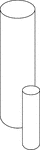2 Similar Cylinders

Illustration of 2 similar cylinders. The height and diameter of the smaller cylinder is half that of…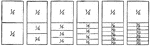Fraction Comparisons

Rectangles comparing fraction values to one half: 1/4, 1/6, 1/8, 1/10, and 1/12.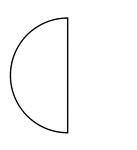Half of a Fraction Pie

One half of a circle (vertical left).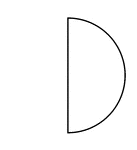Half of a Fraction Pie

One half of a circle (vertical right).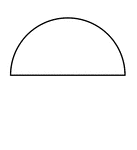Half of a Fraction Pie

One half of a circle (horizontal top).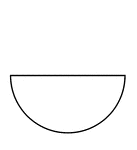Half of a Fraction Pie

One half of a circle (horizontal bottom).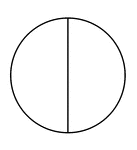Two Halves of a Fraction Pie

A circle divided in half vertically.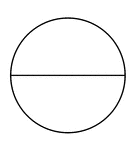Two Halves of a Fraction Pie

A circle divided in half horizontally.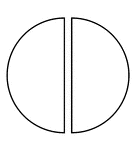Two Halves of a Fraction Pie

A circle divided in half vertically separated.Two Halves of a Fraction Pie

A circle divided in half horizontally separated.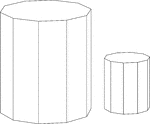Similar Decagonal Prisms

Illustration of 2 similar right decagonal prisms. Both have regular decagons for bases and rectangular…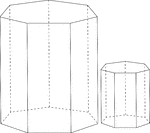Similar Heptagonal/Septagonal Prisms

Illustration of 2 similar right heptagonal/septagonal prisms. Both have regular heptagons/septagons…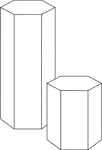Similar Hexagonal Prisms

Illustration of 2 similar right hexagonal prisms. The height of the prism and length of the side of…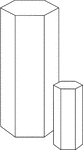Similar Hexagonal Prisms

Illustration of 2 similar right hexagonal prisms. The height of the prism and length of the side of…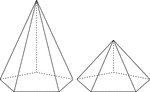2 Right Pentagonal Pyramids

Illustration of 2 right pentagonal pyramids with hidden edges shown. The pentagonal bases are congruent,…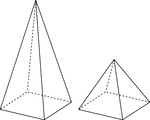2 Right Rectangular Pyramids

Illustration of 2 right rectangular pyramids with hidden edges shown. The rectangular bases are congruent,…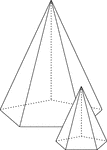Similar Pentagonal Pyramids

Illustration of 2 similar right pentagonal pyramids with hidden edges shown. The height of the pyramid…